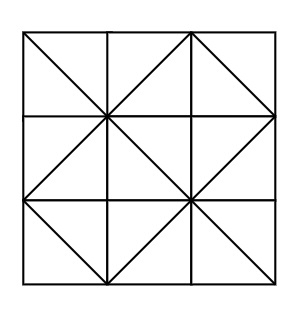# Program to find where the ball lands in a grid box in Python

Suppose, we are given a m x n grid box, where each cell has a board that is positioned either from the top-right to bottom-left, or from the top-left to the bottom-right. Now from the top cells, a ball is put into the box and we have to check if that ball reaches the bottom of the box. The grid is given as a matrix. If a cell is marked 1 the diagonal board spans from the top-left to the bottom-right; if it is marked -1 it spans from the top-right to the bottom-left corner. If n balls are put into the box, we have to find out how many balls reach the bottom.Example of a 3x3 grid box.

So, if the input is like mat =

111-1
-111-1
1-1-11
1-11-1

then the output will be [-1, -1, -1, -1]

To solve this, we will follow these steps −

• i := number of rows in mat

• j := number of columns in mat

• res := a new list

• for val in range 0 to j, do

• x := val

• for r in range 0 to i, do

• s := mat[r, x]

• x := x + mat[r, x]

• if x < 0 or x >= j or mat[r, x] is not same as s, then

• add element -1 at the end of res

• come out from the loop

• otherwise,

• add x at the end of res

• return res

## Example

Let us see the following implementation to get better understanding

def solve(mat):
i, j = map(len, (mat, mat))
res = []
for val in range(j):
x = val
for r in range(i):
s = mat[r][x]
x += mat[r][x]
if x < 0 or x >= j or mat[r][x] != s:
res += [-1]
break
else:
res += [x]
return res

print(solve([[1, 1, 1, -1], [-1, 1, 1, -1], [1, -1, -1, 1],[1, -1, 1, -1] ]))

## Input

[[1, 1, 1, -1], [-1, 1, 1, -1], [1, -1, -1, 1],[1, -1, 1, -1] ]


## Output

[-1, -1, -1, -1]